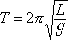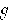The period T of a simple pendulum depends on the length L of the pendulum and the gravitational force constantof the planet or moon whose surface the pendulum is near.

Note: Unless otherwise specified, one should assume the pendulum to be near the surface of the earth.

Wow! The period of a simple pendulum does not depend on the mass of the bob.

Pitfall Avoidance Note: In solving simple pendulum problems, don't use any expressions involving the symbol k (lower case k). That k is the force constant for a spring. There is no spring in the case of a simple pendulum.